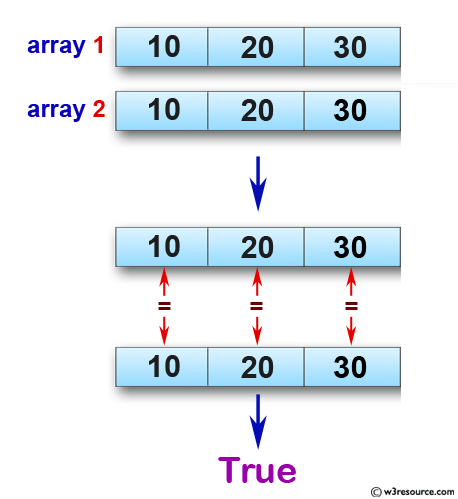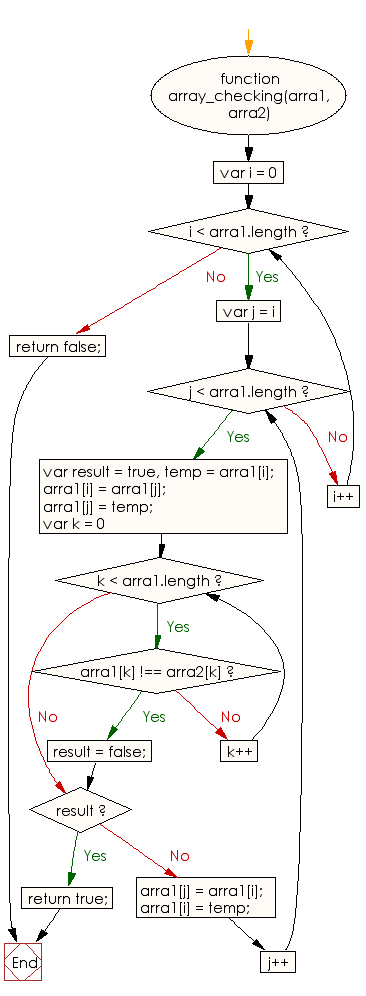# JavaScript: Check whether two arrays of integers of same length are similar or not

## JavaScript Basic: Exercise-87 with Solution

Write a JavaScript program to check whether two arrays of integers of same length are similar or not. The arrays will be similar if one array can be obtained from another array by swapping at most one pair of elements.

Pictorial Presentation:Sample Solution:

HTML Code:

``````<!DOCTYPE html>
<html>
<meta charset="utf-8">
<meta name="viewport" content="width=device-width">
<title>Write a JavaScript program to check whether two arrays of integers of same length are similar or not. The arrays will be similar if one array can be obtained from another array by swapping at most one pair of elements</title>
<body>

</body>
</html>
```
```

JavaScript Code:

``````function array_checking(arra1, arra2) {

for(var i = 0; i < arra1.length; i++) {
for(var j = i; j < arra1.length; j++) {
var result = true,
temp = arra1[i];
arra1[i] = arra1[j];
arra1[j] = temp;
for(var k = 0; k < arra1.length; k++) {
if(arra1[k] !== arra2[k]) {
result = false;
break;
}
}
if(result) {
return true;
}
arra1[j] = arra1[i];
arra1[i] = temp;
}
}
return false;
}

console.log(array_checking([10,20,30], [10,20,30]))
console.log(array_checking([10,20,30], [30,10,20]))
console.log(array_checking([10,20,30,40], [10,30,20,40]))
```
```

Sample Output:

```true
false
true
```

Flowchart:ES6 Version:

``````function array_checking(arra1, arra2) {

for(let i = 0; i < arra1.length; i++) {
for(let j = i; j < arra1.length; j++) {
let result = true;
const temp = arra1[i];
arra1[i] = arra1[j];
arra1[j] = temp;
for(let k = 0; k < arra1.length; k++) {
if(arra1[k] !== arra2[k]) {
result = false;
break;
}
}
if(result) {
return true;
}
arra1[j] = arra1[i];
arra1[i] = temp;
}
}
return false;
}

console.log(array_checking([10,20,30], [10,20,30]))
console.log(array_checking([10,20,30], [30,10,20]))
console.log(array_checking([10,20,30,40], [10,30,20,40]))
``````

Live Demo:

See the Pen javascript-basic-exercise-87 by w3resource (@w3resource) on CodePen.

What is the difficulty level of this exercise?

Test your Programming skills with w3resource's quiz.

﻿

## JavaScript: Tips of the Day

Converts a string to title case

Example:

```const toTitleCase = str =>
str
.match(/[A-Z]{2,}(?=[A-Z][a-z]+[0-9]*|\b)|[A-Z]?[a-z]+[0-9]*|[A-Z]|[0-9]+/g)
.map(x => x.charAt(0).toUpperCase() + x.slice(1))
.join(' ');
console.log(toTitleCase('some_database_field_name')); // 'Some Database Field Name'
console.log(toTitleCase('Some label that needs to be title-cased')); // 'Some Label That Needs To Be Title Cased'
console.log(toTitleCase('some-package-name')); // 'Some Package Name'
console.log(toTitleCase('some-mixed_string with spaces_underscores-and-hyphens')); // 'Some Mixed String With Spaces Underscores And Hyphens'
```

Output:

```"Some Database Field Name"
"Some Label That Needs To Be Title Cased"
"Some Package Name"
"Some Mixed String With Spaces Underscores And Hyphens"
```